V.5 No 2 19 On light aberration2. Classical description of aberration of light It is known that “all stationary stars located near the axis of Earth orbit describe during a year a circular orbit with the diameter of 41"; this is the hodograph of our Earth’s orbit. We can also observe two stationary stars near the plane of the Earth orbit, one in radial and another in tangential direction. Their angular distance also changes during a year by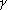= 20,5 seconds, but their path seems to be a direct line. The stars located between the axis and plane of the Earth orbit describe during the year the elliptic orbits with the big axis equal to 41". The whole phenomenon is called astronomical aberration. It was discovered by Bradley and explained (by Bradley - Authors) in 1728. The relation u/c, i.e. the relation of Earth orbital velocity to that of light, astronomers call ‘the aberration constant’ “ [4, p. 193]. “The classical theory of light aberration, based on the idea of light propagation in the stationary medium - ether, brings us to the following kind of regularity in aberration shift of a celestial body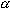(along the big circle of celestial sphere that goes through the body and apex - the point toward which the observer moves, - toward the apex), the angle between directions to the body and to the apex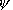and the velocity of observer v (? is the speed of light):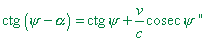(1)
 [5, p. 9]. “If we denote the angle between the direction in which we see the body and the direction to the apex as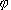,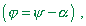(2)
 we can record (1) as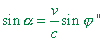(3)
 [5, p. 10]. In Fig. 1 we can see all mentioned angles.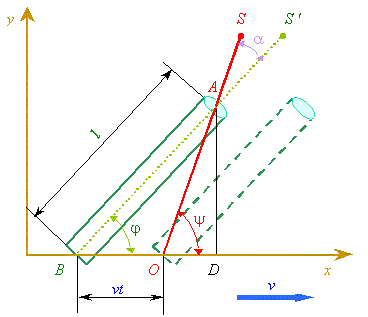Fig. 1. The graph to calculate the classical effect of light aberration in case when the observer moved with some velocity v relatively the distanced stationary source
 Fig. 1 is grounded on the basic regularity that defines the phenomenology of aberration phenomenon. As we can see from the construction, this regularity is connected not with mutually moving reference frames but with the fact that in order to register the direction towards the star, we anyway use the directed section - usually the tube of telescope, rulers of triquetra or quadrant either of the dioptric tube of universal quadrant, or alidade - a rotating ruler with two diopters (see Fig. 2). But in any case this is some directed ruler that determines the angular location of a celestial body by coincidence of its start and finish.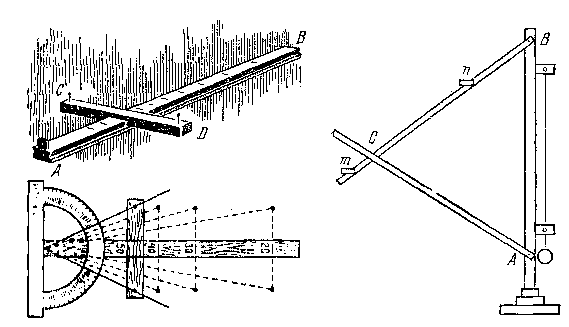a) “the astronomical staff (top left) and triquetra (right). Bottom left is the drawing that explains the action of astronomical staff” [6, p. 8]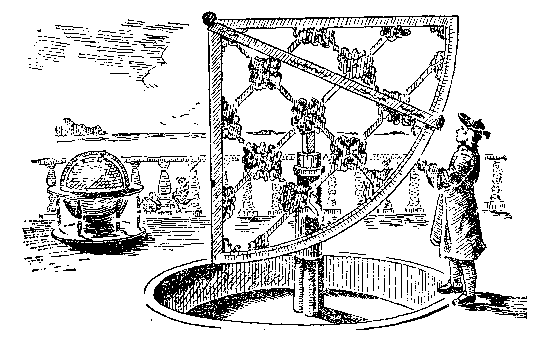b) "ancient quadrant" [6, p. 9]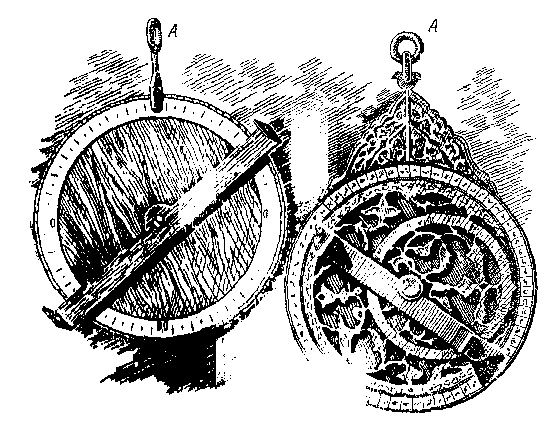c) “ancient (right) and hand-made astrolabe” [6, p. 10]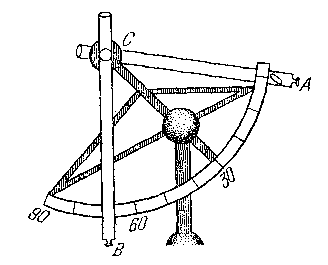d) “universal quadrant” [6, p. 11] Fig. 2. Some goniometric astronomic instruments 
 As we see from Fig. 1, “should the telescopic axis (S 'B - Authors) were located in parallel to this direct line (SO - Authors), the light coming into the opening A would not pass through the opening B: B would coincide with O at the moment when light passing through A. But during the light moving along the tube, the opening B would shift rightward from the point O ” [6, p. 155]. Hence, the role of directing ruler is determined by the finite time during which the beam passes the distance along the directed section l uniformly shifting in some direction together with the accompanying reference frame and independently of the beam.

Contents: / 18 / 19 / 20 / 21 / 22 / 23 / 24 / 25 / 26 / 27 / 28 /